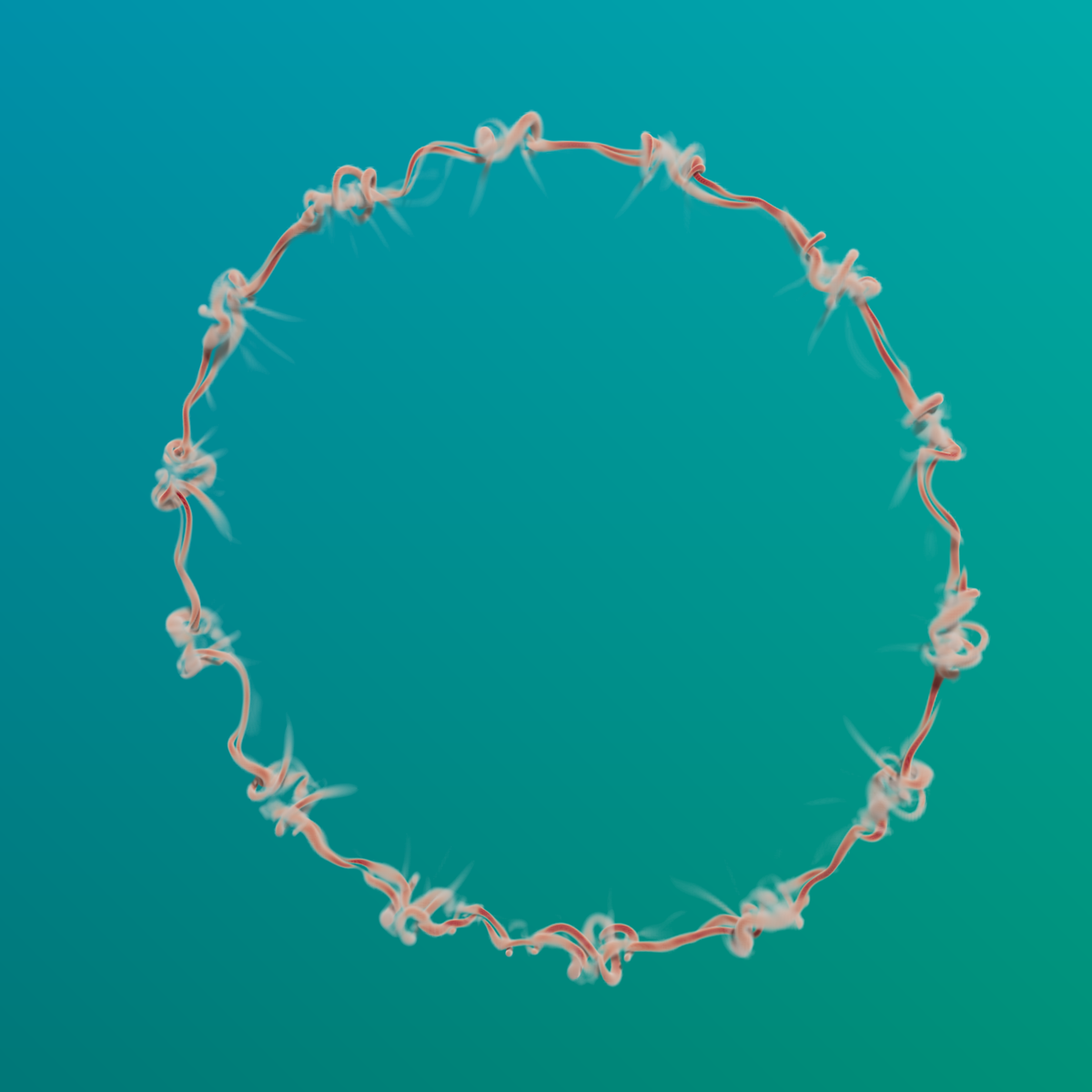# Bwatch usage for visualization of a dump file

The dumpfile that contains a velocity field is generated on a super computer with MPI. We render the data on a local machine, accelerated with openMP.Volumetric rendering inspired by Palas Kumar Farsoiya

Visualization of the \lambda_2 field as volume, and later as iso surface, color coded with the local velocity vector

#include "grid/octree.h"
#include "utils.h"
#include "bwatch.h"
#include "lambda2.h"

scalar dummy[];
vector uc[];

int main() {
//Obtain the dump file from elsewhere
scalar l2[];
lambda2 (uc, l2);
foreach()
l2[] = l2[] > 0 ? 0 : -l2[];
boundary ({l2});
watch (fov = 50, nx = 2400, ny = 2400); //Highres
watch (O = {30, 30., 100});
volume (l2, cols = true, sc = 0.005, mval = 0.001,
min = -0.1, max = 0.1, shading = 1);
store (fopen("smk.ppm", "w"));
plain();
system( "convert smk.ppm -resize 40% rings.png"); //4x MSAA

watch (nx = 900, ny = 900);
FILE * fp = popen ("ppm2mp4 -r 20 mov.mp4", "w");
for (double a = 0; a <= 2*pi; a += 0.025*pi) {
watch (O = {100*sin(a), 30., 100*cos(a)});
volume (l2, sc = 0.01, mval = 0.001, col = {255, 20, 30});
sphere (R = 200, mat = {dull = true, col = {255, 255, 255}});
store (fp);
plain();
}

for (double a = 0; a <= 2*pi; a += 0.025*pi) {
watch (O = {100*sin(a), 30., 100*cos(a)});
equiplane (l2, 0.005, insideout = true,
mat = {v = uc, min = -0.05, max = 0.05});
sphere (R = 200, mat = {dull = true, col = {255, 255, 255}});
store (fp);
plain();
}

pclose(fp);
}